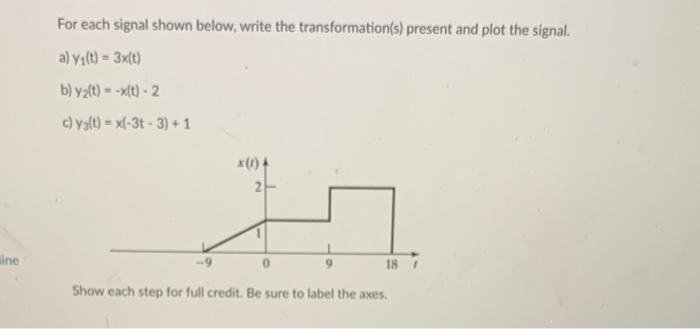Home / Expert Answers / Electrical Engineering / for-each-signal-shown-below-write-the-transformation-s-present-and-plot-the-signal-a-y-1-t-pa789

# (Solved): For each signal shown below, write the transformation(s) present and plot the signal. a) \( y_{1}(t ...For each signal shown below, write the transformation(s) present and plot the signal. a) \( y_{1}(t)=3 x(t) \) b) \( y_{2}(t)=-x(t)-2 \) c) \( y_{3}(t)=x(-3 t-3)+1 \) Show each step for full credit. Be sure to label the axes.

We have an Answer from Expert